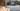# parseInt() 不总是正确地转换为整数

## parseInt() 如何工作

`parseInt()` 有如下定义：

``parseInt(value, radix?)``

``parseInt('0xA'); // 10``

``````parseInt('0xA', 16); // 10

parseInt('A', 16); // 10``````

``````parseInt('010'); // 8

parseInt('0109'); // 8 // 忽略位数 ≥ 8``````

### 例子

``````parseInt('   12', 10); // 12
parseInt('12**', 10); // 12
parseInt('12.34', 10); // 12
parseInt(12.34, 10); // 12``````

``parseInt(1000000000000000000000.5, 10); // 1``

``String(1000000000000000000000.5); // '1e+21'``

``````parseInt(0.0000008, 10); // 8

//同理
String(0.0000008); // '8e-7'``````

``````String(1e20); // '100000000000000000000'
String(1e21); //'1e+21'``````

## 替代 parseInt() 的方法

### Math.trunc()

ES6 中添加 `Math.trunc()` 函数用于截断数字的小数部分：

``````Math.trunc(80.6); // 80
Math.trunc('80.1'); // 80
Math.trunc('hello'); // NaN
Math.trunc(NaN); // NaN
Math.trunc(undefined); // NaN``````

### toInteger()

``````function toInteger(x) {
x = Number(x);
return x < 0 ? Math.ceil(x) : Math.floor(x);
}``````

``````toInteger(3.2); // 3
toInteger(3.5); // 3
toInteger(3.8); // 3

toInteger(-3.2); // -3
toInteger(-3.5); // -3
toInteger(-3.8); // -3``````

### Math.round()

``````Math.round(3.2); // 3
Math.round(3.5); // 4
Math.round(3.8); // 4

Math.round(-3.2); // -3
Math.round(-3.5); // -3
Math.round(-3.8); // -4``````

4 被认为最接近 3.5，而 -3 被认为最接近 -3.5。这与 `parseInt()` 行为不同。